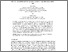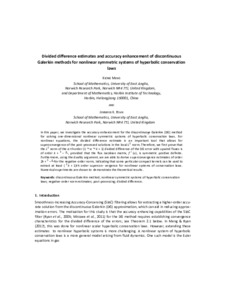# Divided difference estimates and accuracy enhancement of discontinuous Galerkin methods for nonlinear symmetric systems of hyperbolic conservation laws

Meng, Xiong and Ryan, Jennifer K. (2018) Divided difference estimates and accuracy enhancement of discontinuous Galerkin methods for nonlinear symmetric systems of hyperbolic conservation laws. IMA Journal of Numerical Analysis, 38 (1). 125–155. ISSN 0272-4979Preview PDF (Accepted manuscript) - Accepted Version Download (1MB) | Preview

## Abstract

In this paper, we investigate the accuracy-enhancement for the discontinuous Galerkin (DG) method for solving one-dimensional nonlinear symmetric systems of hyperbolic conservation laws. For nonlinear equations, the divided difference estimate is an important tool that allows for superconvergence of the post-processed solutions in the local L2-norm. Therefore, we first prove that the L2-norm of the α-th order (1≤ α≤ k+1) divided difference of the DG error with upwind fluxes is of order k+(3-α)/2, provided that the flux Jacobian matrix, f'(u), is symmetric positive definite. Furthermore, using the duality argument, we are able to derive superconvergence estimates of order 2k+(3-α)/2 for the negative-order norm, indicating that some particular compact kernels can be used to extract at least (3k/2+1)-th order superconvergence for nonlinear systems of conservation laws. Numerical experiments are shown to demonstrate the theoretical results.

Item Type: Article discontinuous galerkin,nonlinear symmetric systems of hyperbolic conservation,negative-order norm estimates,post-processing,divided difference Faculty of Science > School of Mathematics Faculty of Science > Research Groups > Fluid and Solid Mechanics https://academic.oup.com/imajna/article/... Pure Connector 09 Dec 2016 00:06 21 Oct 2022 08:30 https://ueaeprints.uea.ac.uk/id/eprint/61665 10.1093/imanum/drw072View Item